### Father Robert Spitzer: The Anthropic Principle - Theistic Implications

Constants and Ratios

 Boltzmann’s constant k = 1.38 x 10-23 J/oK Planck’s constant ħ = 6.63 z 10-34 J/s Speed of light c = 3.00 x 108 m/s Gravitational constant G = 6.67 x 10-11 (N - m2)/kg Weak force constant gw = 1.43 x 10-62 (SI units) Strong force constant gs = 15 (SI units) Hubble constant H = 2 x 10-18 (SI units) Cosmological constant L = <10-53 (SI units) Cosmic photon/proton ratio S = 109 (SI units) Permittivity of free space e = 8.85 x 10-12 (SI units) Rest mass of a neutron Mn = 1.674 x 10-27 kg Rest mass of an electron Me = 9.11 x 10-31 kg Rest mass of a proton Mp = 1.672 x 10-27 kg Electron or proton unit charge e = 1.6 x 10-19 coul Mass-energy relation c2 = (E/m) J/kg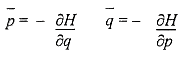Hamilton’s Equations for Mechanical Interaction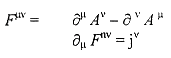Maxwell’s Equations for Electrodynamic interactions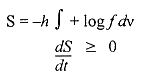Boltzmann’ s Equations for Statistical Mechanics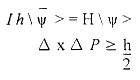Schrodinger’ s Equations for Quantum Mechanical Interaction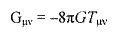Einstein’s Equation for the General Theory at Relativity (Regulating the interaction at mass energy with space - time coordinate structure)

 Email link | Feedback | Contributed by: The Discovery Institute

Father Robert Spitzer: The Anthropic Principle - Theistic Implications

#### Source:

Presented at the 'Cosmos and Creator'conference sponsored by the C. S. Lewis Institute and the Discovery Institute